# Digital flip flop circuits explained – Learn about flip-flops and how to trigger them.

## Introduction

In this article let us see what flip flops are and how they are used in digital circuits.

Basically the logic circuits are divided into

• Combinational logic circuits

• Sequential logic circuits

In combinational logic circuits, the output at any instant of time depends only on the inputs present at that time.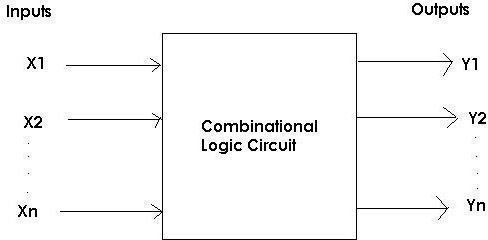But there are circuits in which the output at any instant of time depends not only on the present input of the system but also on the past outputs obtained by the system. Such logic circuits are called sequential logic circuits.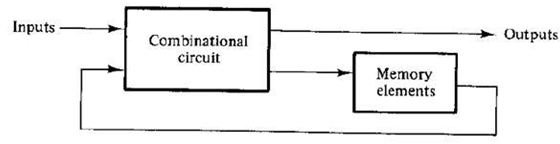Now let us understand flip-flops, their importance and uses in the sequential circuits.

## What are Flip-Flops?

We know that sequential logic circuit depends also on the past output of the system. So they require a memory element to store the past outputs. For this purpose the flip-flops are used.

Flip-flops are nothing but the memory elements used in the sequential circuits for storing the past output of the system. A flip-flop circuit can maintain a binary state indefinitely until directed by any input signal to switch states.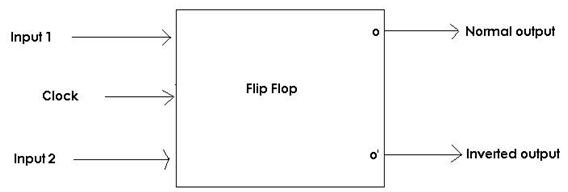A flip-flop circuit normally consists of two inputs, a clock generator (or) clocking signal and two outputs. One being the normal value of the output and the other is the complement value of the bit stored in it. And the output of flip-flop is either logic 0 or logic 1.

A flip-flop has two stable states and hence it is also called as bistable multi-vibrator.

## Difference between Flip-Flop and Latch

Flip-Flop and Latch are the two basic building blocks of a sequential circuit. But there is a subtle difference between the two.

A flip-flop continuously checks its inputs and correspondingly changes its output only at times determined by clocking signal. Where as a latch is a device which continuously checks all its input and correspondingly changes its output, independent of the time determined by clocking signal.

A unique signal called "enable" is provided with latch. The output changes only when the enable signal is active. No change in output takes place when the enable signal is inactive.

## Triggering Of Flip-Flops

We already know that a flip-flop has two stable states. A flip-flop changes from one state to another and comes back to its original state. Now how can we change the state of the flip-flop? The state of the flip-flop can be changed by applying a momentary change to the Input signal. This momentary change is called triggering of a flip-flop. There are clocked flip-flops which are triggered by the pulse generated by clocking signal. A clock pulse is usually a square wave which has 0 as its initial value and goes momentarily to 1 and returns back to 0.

Triggering of flip-flops can be classified into:

• Level Triggering

• Edge Triggering

Level Triggering:

Level triggered flip-flops are dependent on the period of the pulse applied to it. It is further classified into two types.

• Positive Level Triggering

• Negative Level Triggering

If a flip-flop changes its state when the clock pulse applied is positive then it is called as positive level triggering.

On the contrary, if the flip-flop changes its state when the clock pulse applied is negative then it is called negative level triggering.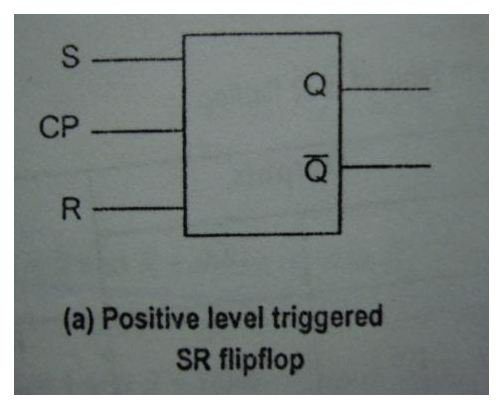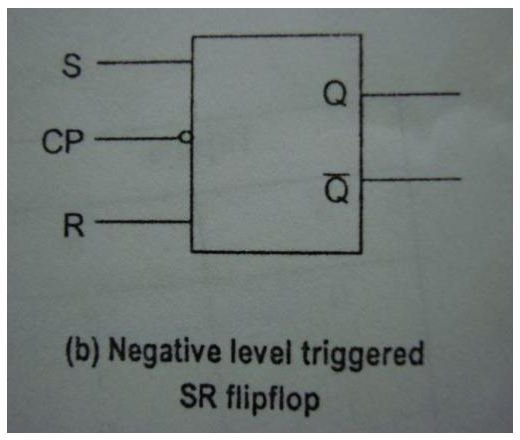Edge Triggering:

The edge triggered flip flops changes state either at positive edges or negative edges of the clock pulse applied and accordingly they are classified into Positive edge triggered Flip flops and Negative edge triggered flip flops. A positive or rising transition is achieved from positive edge flip flops and negative or falling transition is achieved from negative edge flip flops.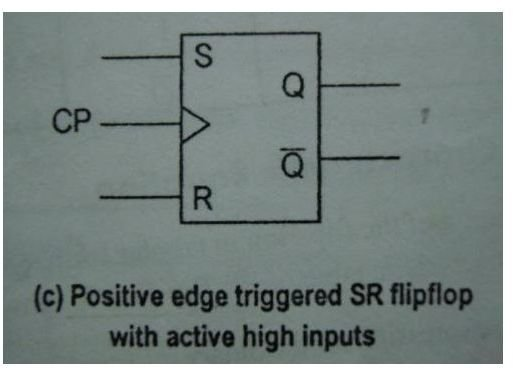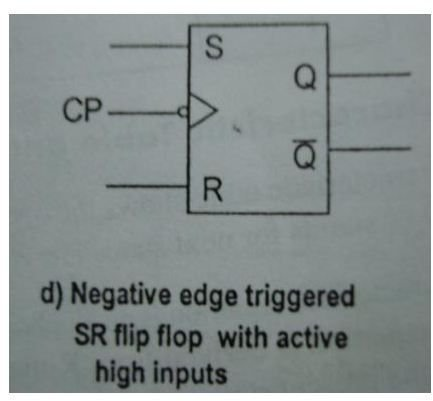In the next article let us discuss the basic circuit of flip-flop and types of flip-flops that are being used in digital circuits for various applications.

## Image and Content Credits

“Digital Electronics” by P.S. Manoharan